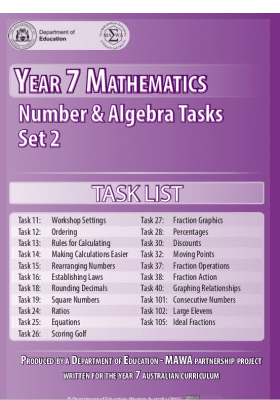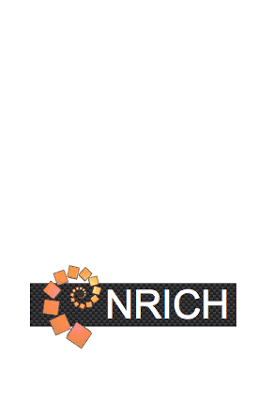Resources for Year 7 students

Resources for Year 7 students

Resources are available across all year levels and learning areas, to give children and young people the best opportunity to continue to learn at home.

Resources

Resources

•Number and Algebra - Addition and subtraction with related denominators

Retrieving data. Wait for a few seconds, then try cutting or copying again.

•Number and Algebra - Addition and subtraction with unrelated denominators

•Number and Algebra - Commutative law and subtraction and division

•Number and Algebra - Commutative law for addition

•Number and Algebra - Commutative law for multiplication

•Number and Algebra - Dividing fractions

•Number and Algebra - Equivalent fractions and the use of the number line

•Number and Algebra - Laws of arithmetic

•Number and Algebra - Multiplication and division of decimals

•Number and Algebra - Multiplying and dividing mixed numerals

•Number and Algebra - Multiplying fractions

•Number and Algebra - Notation

•Number and Algebra - Pronumerals

•Number and Algebra - Square roots and square numbers

•Number and Algebra Task List - Set 1

•Number and Algebra Task List - Set 2

•Number and Algebra Task List - Set 3

•Number and Algebra- Expressing one quantity as a fraction of a second

Read the information about expressing one quantity as a fraction of a second and answer the questions.

•Number and Algebra- Rounding decimals

•Orange drink

A problem solving task exploring proportional reasoning

— 20 Items per Page
Showing 41 - 60 of 90 results.